0
• 聊天消息
• 系统消息
• 评论与回复• 下载海量资料
• 学习在线课程
• 观看技术视频
• 写文章/发帖/加入社区

• 发文章

• 发资料

• 发帖

• 提问

• 发视频

3天内不再提示

#使用Python可视化数据,机器人开发编程

zhKF_jqr_AI 来源：未知 作者：龚婷 2018-03-15 16:56 次阅读

1. 数据集

import numpy as np

import pandas as pd

pd.options.display.max_columns = 12

# 禁用Anaconda警告

import warnings

warnings.simplefilter('ignore')

# 在Jupyter Notebook内部显示图形

%matplotlib inline

import matplotlib.pyplot as plt

# 我们将使用Seaborn库

import seaborn as sns

sns.set()

# SVG格式的图像更清晰

%config InlineBackend.figure_format = 'svg'

# 增加默认的绘图尺寸

from pylab import rcParams

rcParams['figure.figsize'] = 5, 4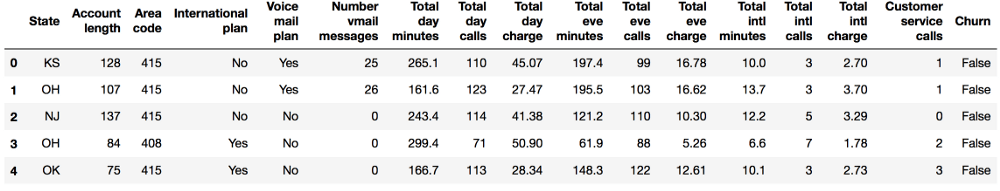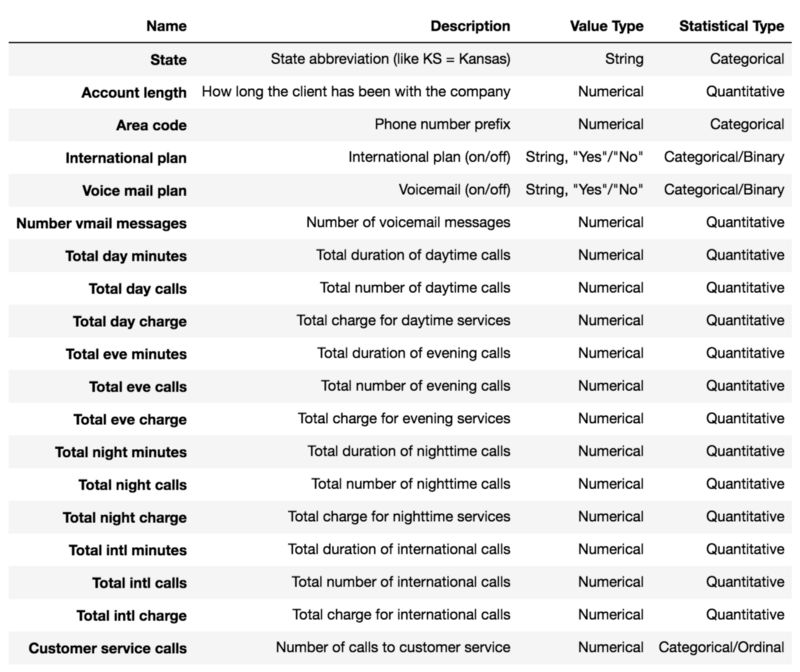2. 单变量可视化

2.1 数量特征

features = ['Total day minutes', 'Total intl calls']

df[features].hist(figsize=(12, 4));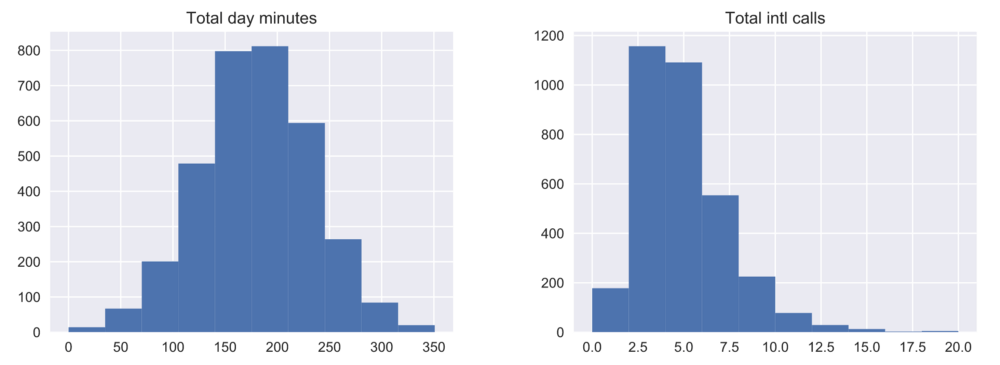df[features].plot(kind='density', subplots=True,

layout=(1, 2), sharex=False, figsize=(12, 4));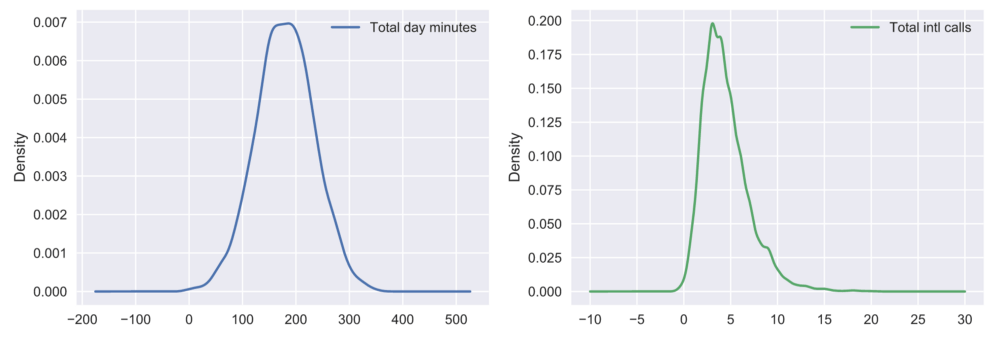sns.distplot(df['Total intl calls']);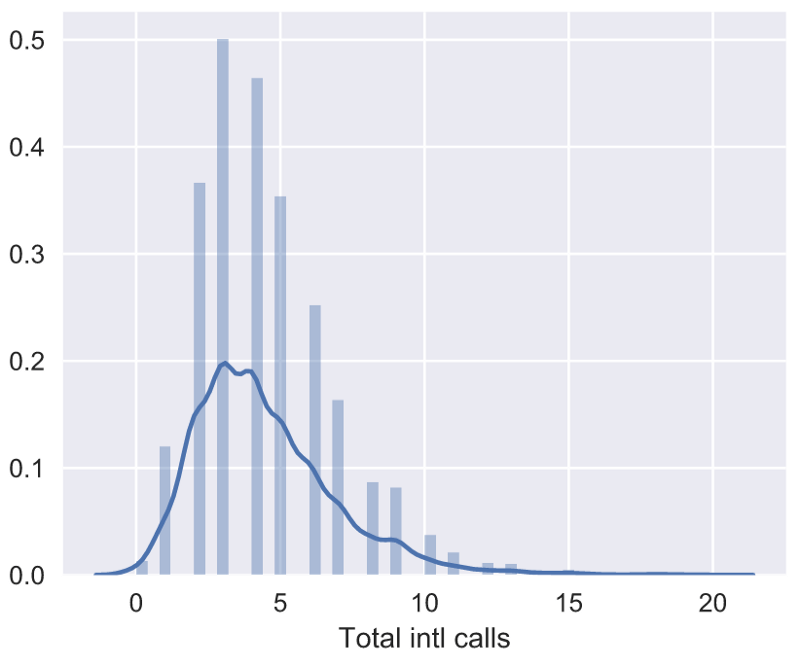_, ax = plt.subplots(figsize=(3, 4))

sns.boxplot(data=df['Total intl calls'], ax=ax);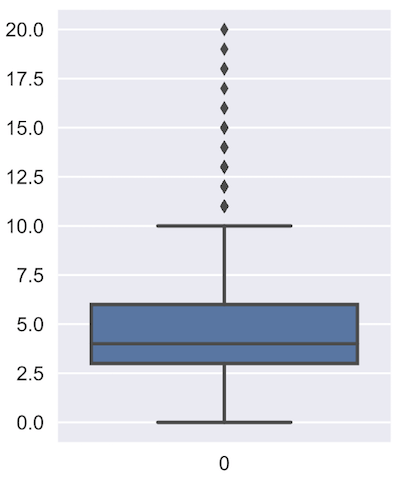_, axes = plt.subplots(1, 2, sharey=True, figsize=(6, 4))

sns.boxplot(data=df['Total intl calls'], ax=axes); sns.violinplot(data=df['Total intl calls'], ax=axes);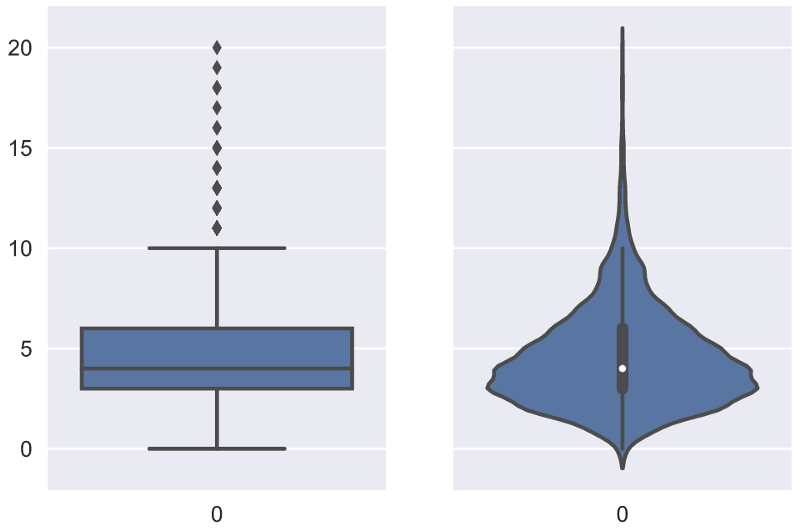describe()

df[features].describe()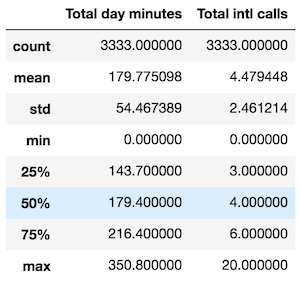describe()的输出基本上是自解释性的。

2.2 类别和二元特征

df['Churn'].value_counts()

False 2850

True 483

Name: Churn, dtype: int64

_, axes = plt.subplots(nrows=1, ncols=2, figsize=(12, 4))

sns.countplot(x='Churn', data=df, ax=axes);

sns.countplot(x='Customer service calls', data=df, ax=axes);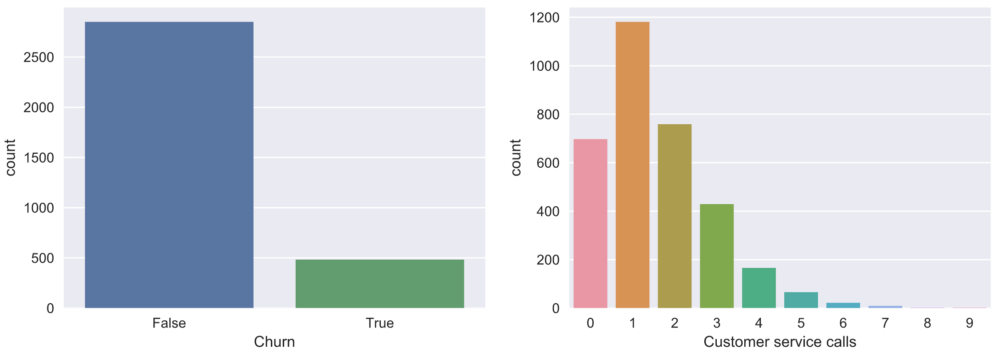3. 多变量可视化

3.1 数量——数量

# 丢弃非数值变量

numerical = list(set(df.columns) -

set(['State', 'International plan',

'Voice mail plan', 'Area code', 'Churn',

'Customer service calls']))

# 计算和绘图

corr_matrix = df[numerical].corr()

sns.heatmap(corr_matrix);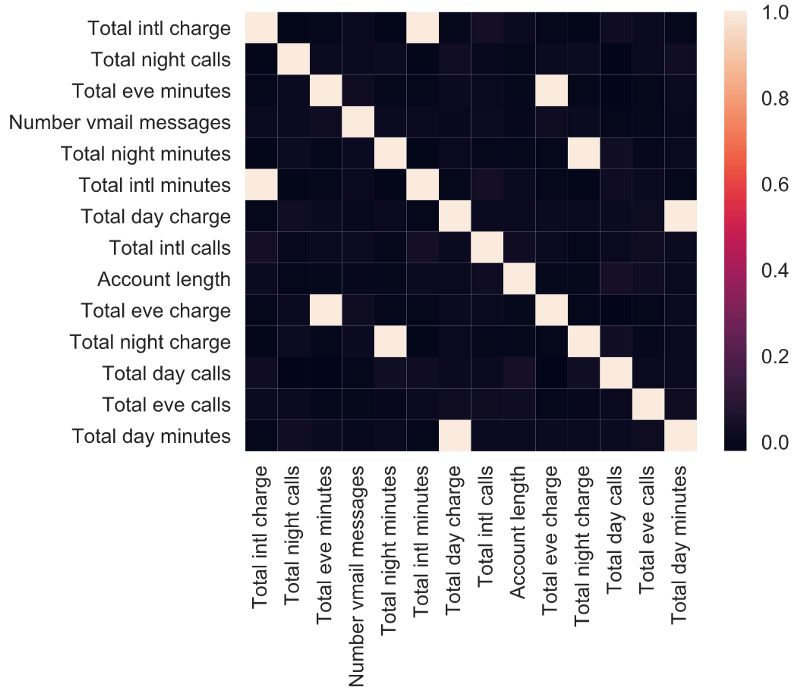numerical = list(set(numerical) -

set(['Total day charge', 'Total eve charge',

'Total night charge', 'Total intl charge']))

plt.scatter(df['Total day minutes'], df['Total night minutes']);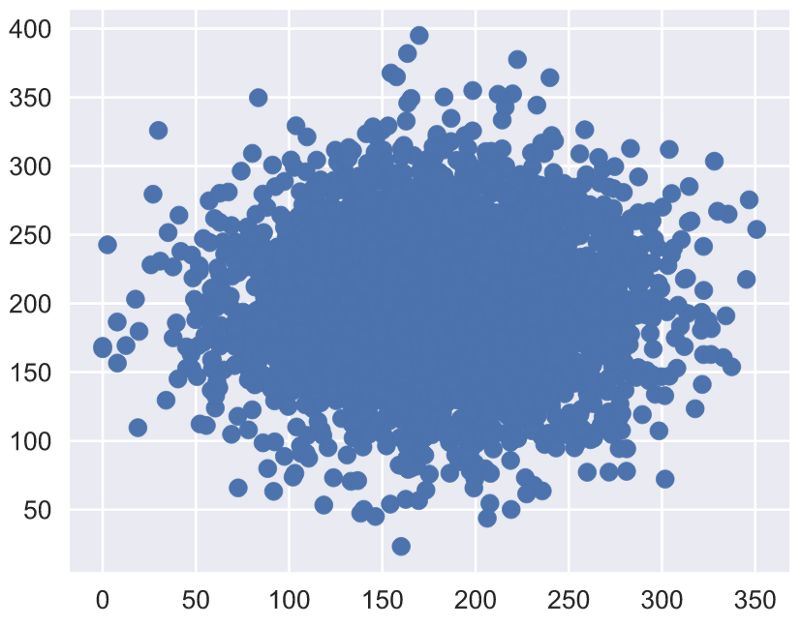seaborn库创建的散点图有一个略微奇特的选项：

sns.jointplot(x='Total day minutes', y='Total night minutes',

data=df, kind='scatter');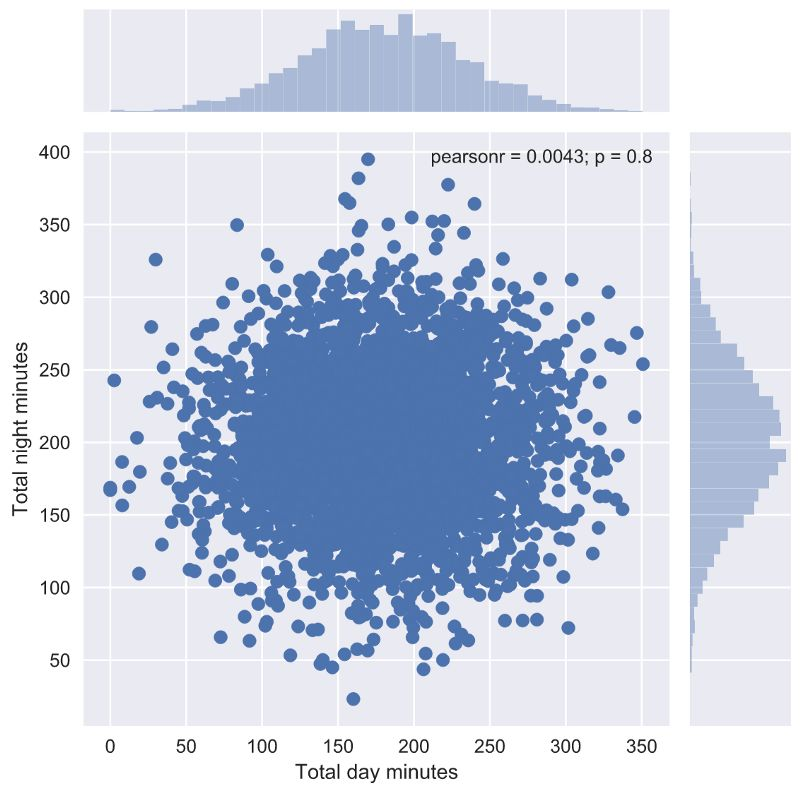jointplot()函数绘制了两张直方图，某些情形下它们可能会有用。这一函数还可以让我们绘制平滑过的joint plot：

sns.jointplot('Total day minutes', 'Total night minutes',

data=df, kind="kde", color="g");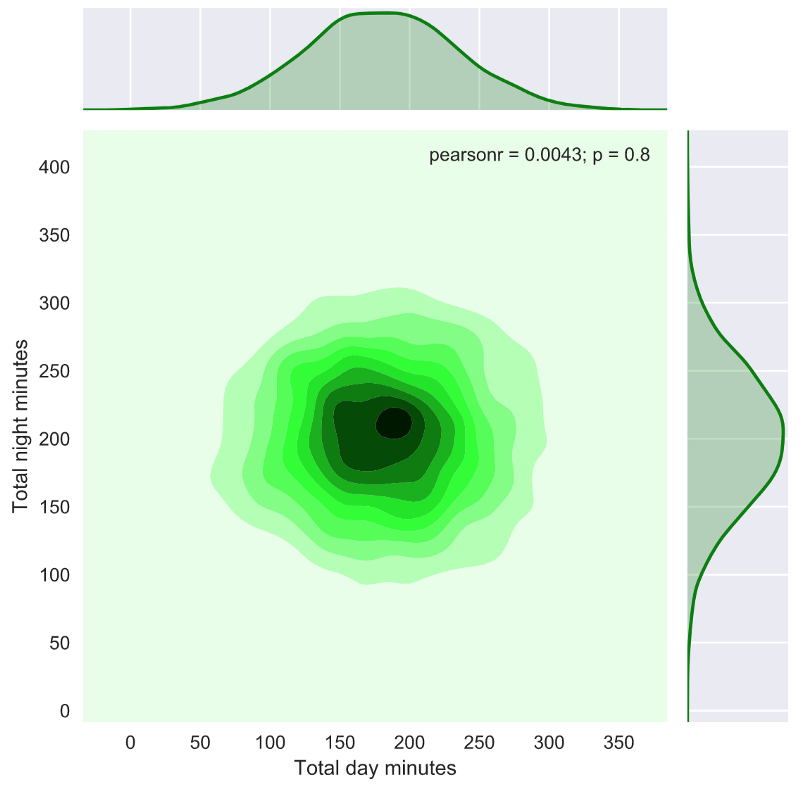# 使用SVG格式可能导致pairplot变得非常慢

%config InlineBackend.figure_format = 'png'

sns.pairplot(df[numerical]);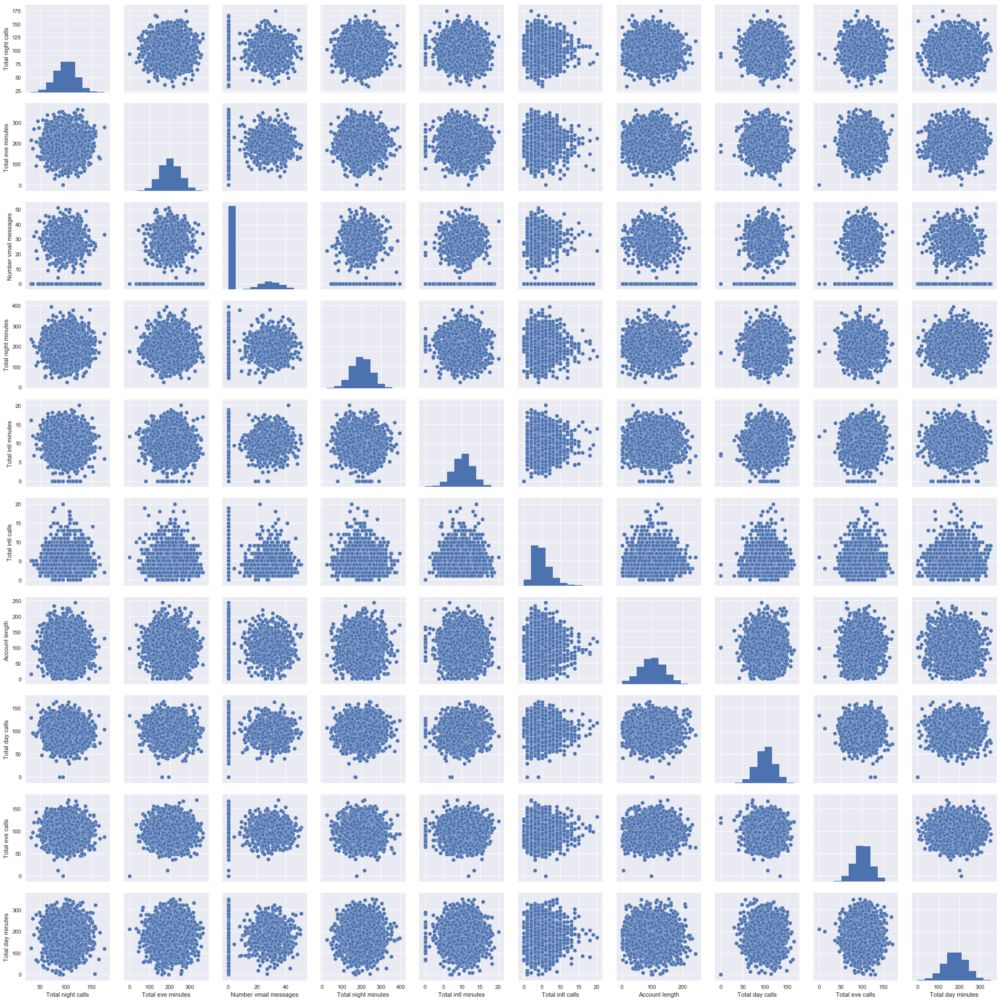3.2 数量——类别

sns.lmplot('Total day minutes', 'Total night minutes', data=df,

hue='Churn', fit_reg=False);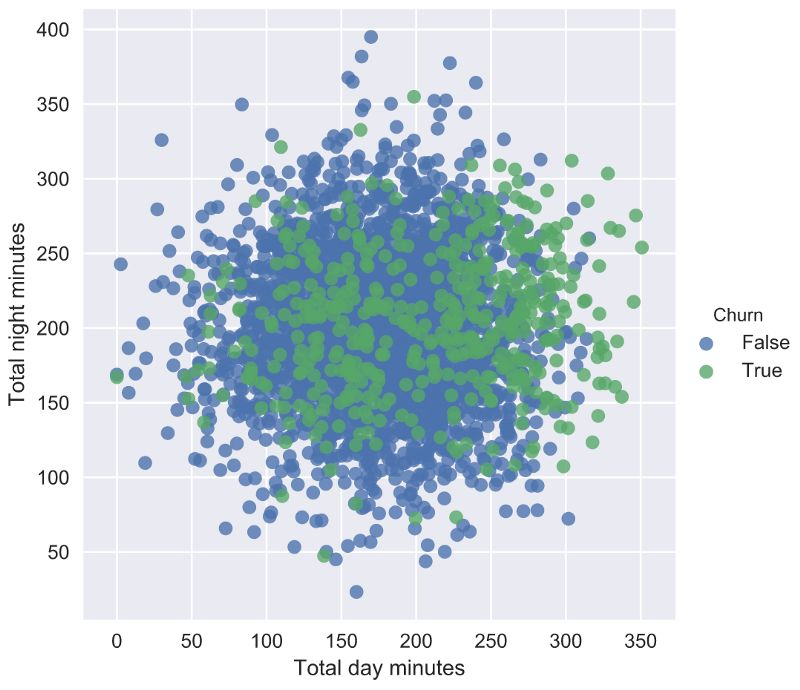# 有时我们可以将有序变量作为数值变量分析

numerical.append('Customer service calls')

fig, axes = plt.subplots(nrows=3, ncols=4, figsize=(10, 7))

for idx, feat in enumerate(numerical):

ax = axes[int(idx / 4), idx % 4]

sns.boxplot(x='Churn', y=feat, data=df, ax=ax)

ax.set_xlabel('')

ax.set_ylabel(feat)

fig.tight_layout();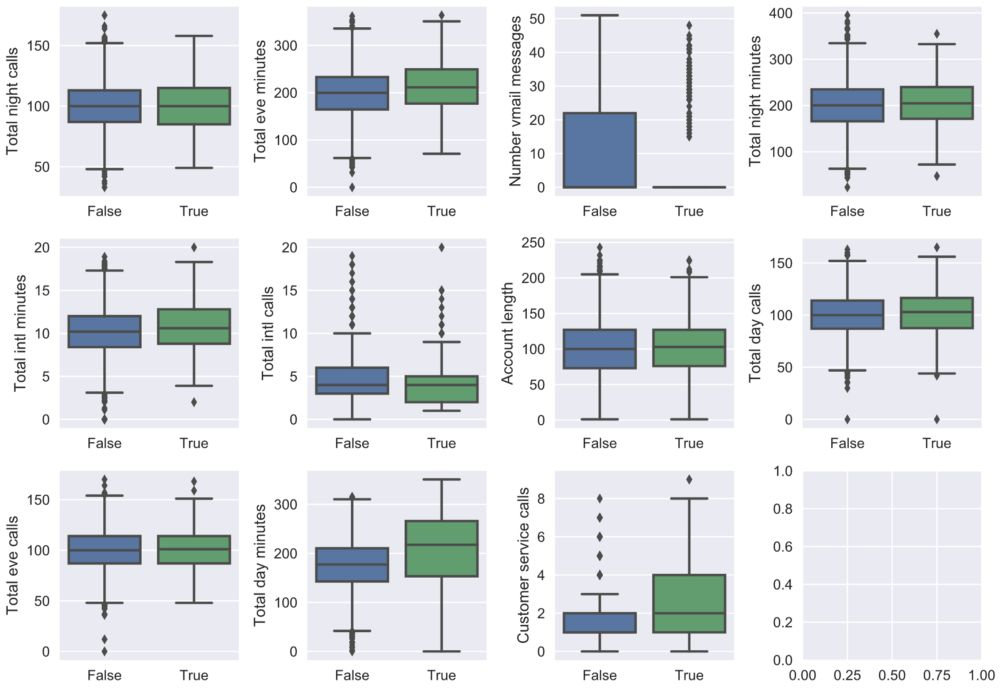_, axes = plt.subplots(1, 2, sharey=True, figsize=(10, 4))

sns.boxplot(x='Churn', y='Total day minutes',

data=df, ax=axes);

sns.violinplot(x='Churn', y='Total day minutes',

data=df, ax=axes);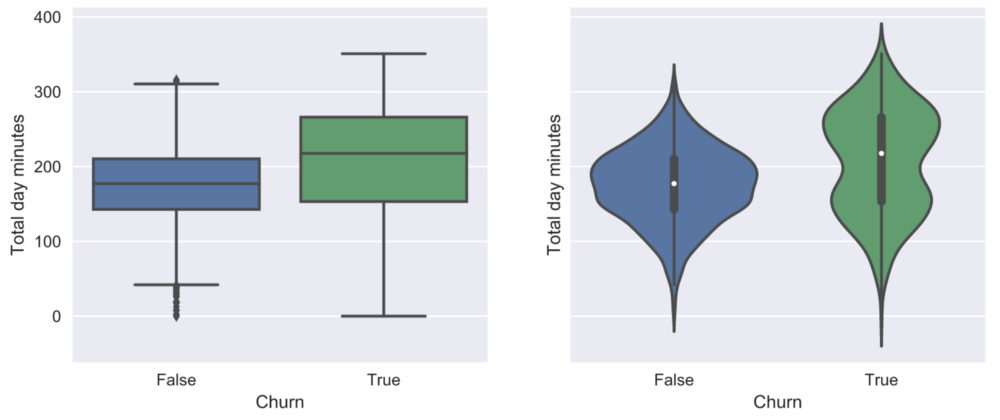sns.factorplot(x='Churn', y='Total day minutes',

col='Customer service calls',

data=df[df['Customer service calls'] < 8],

kind="box", col_wrap=4, size=3, aspect=.8);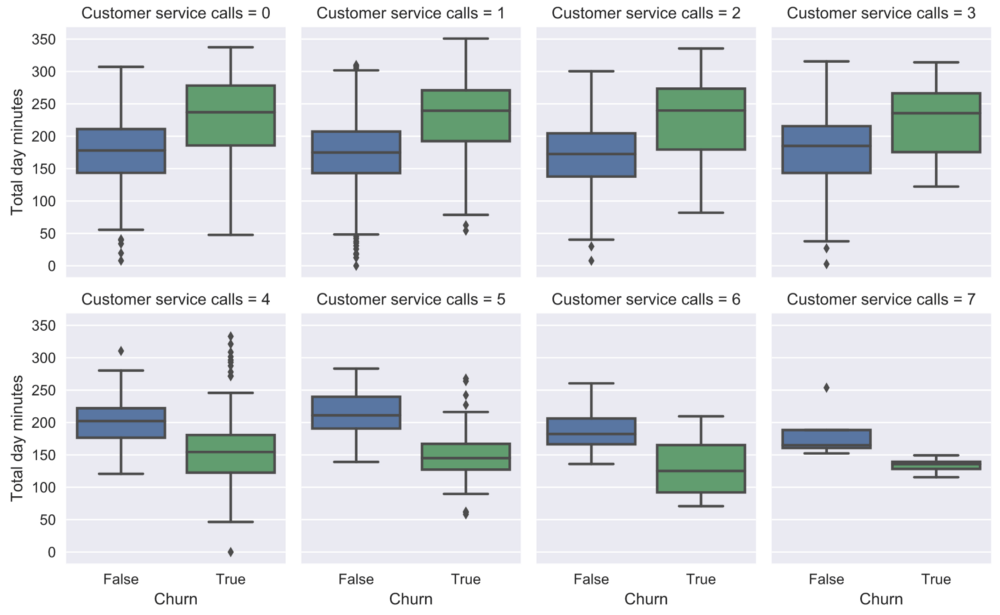3.3 类别——类别

sns.countplot(x='Customer service calls', hue='Churn', data=df);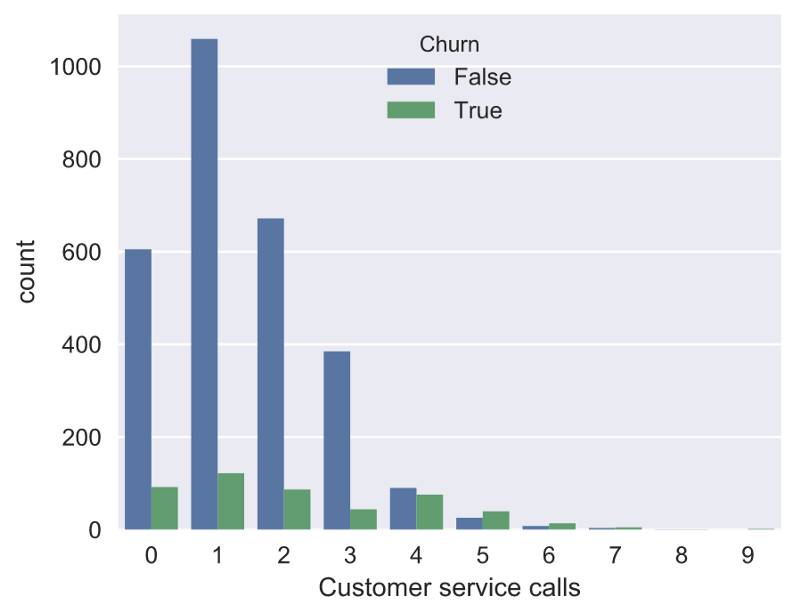_, axes = plt.subplots(1, 2, sharey=True, figsize=(10, 4))

sns.countplot(x='International plan', hue='Churn',

data=df, ax=axes);

sns.countplot(x='Voice mail plan', hue='Churn',

data=df, ax=axes);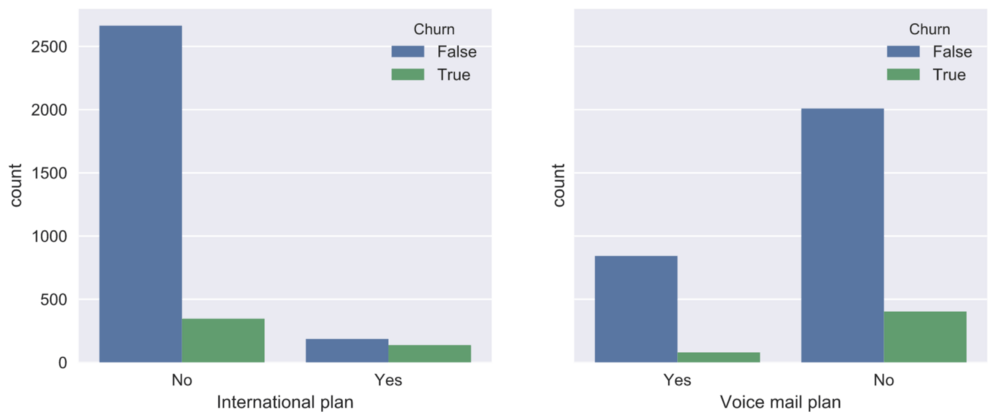pd.crosstab(df['State'], df['Churn']).T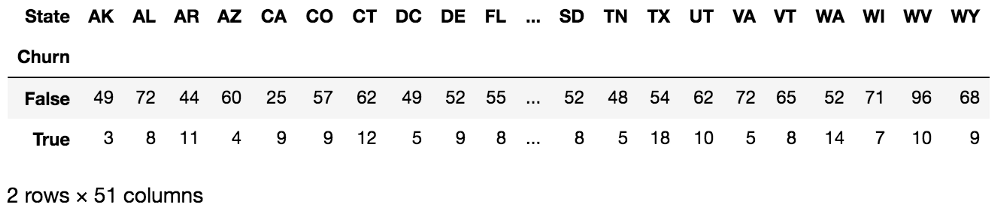State（州）的不同值很多：51. 我们看到每个周只有少量数据点——每个州只有3到17个客户抛弃了运营商。让我们暂时忽略这一点，计算每个州的离网率，由高到低排列：

df.groupby(['State'])['Churn'].

agg([np.mean]).

sort_values(by='mean', ascending=False).T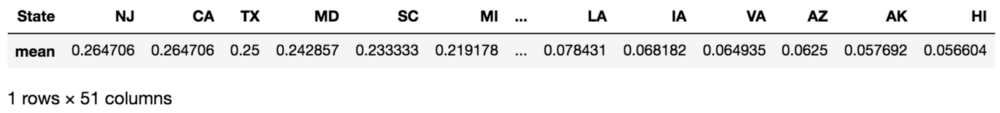4. 全数据集

4.1 幼稚方法

4.2 降维

4.3 t-SNE

from sklearn.manifold import TSNE

from sklearn.preprocessing importStandardScaler

X = df.drop(['Churn', 'State'], axis=1)

X['International plan'] = X['International plan'].

map({'Yes': 1, 'No': 0})

X['Voice mail plan'] = X['Voice mail plan'].

map({'Yes': 1, 'No': 0})

scaler = StandardScaler()

X_scaled = scaler.fit_transform(X)

tsne = TSNE(random_state=17)

tsne_repr = tsne.fit_transform(X_scaled)

plt.scatter(tsne_repr[:, 0], tsne_repr[:, 1]);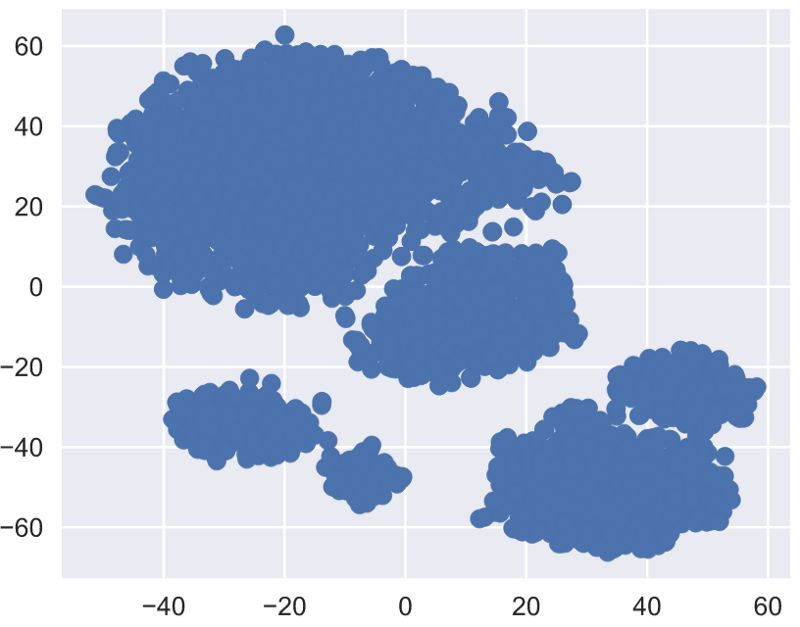plt.scatter(tsne_repr[:, 0], tsne_repr[:, 1],

c=df['Churn'].map({False: 'green', True: 'red'}));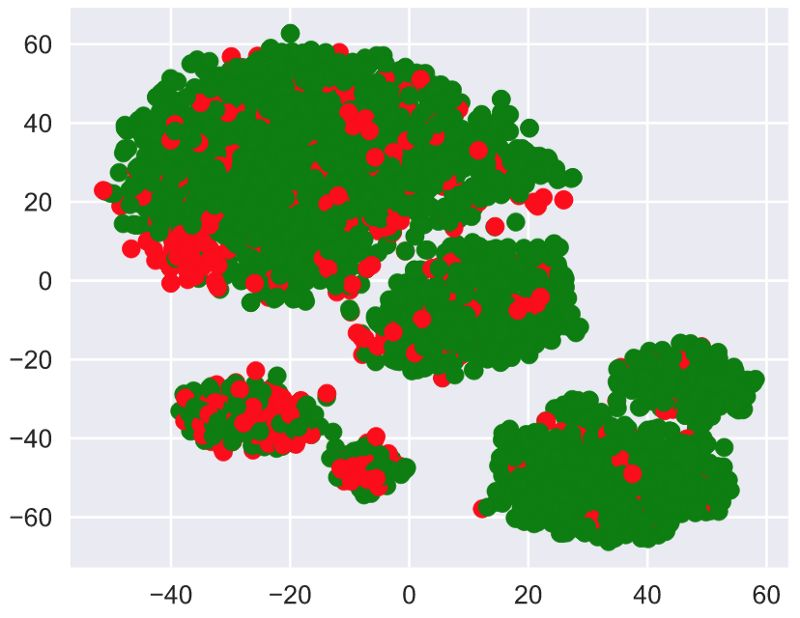_, axes = plt.subplots(1, 2, sharey=True, figsize=(12, 5))

for i, name in enumerate(['International plan', 'Voice mail plan']):

axes[i].scatter(tsne_repr[:, 0], tsne_repr[:, 1],

c=df[name].map({'Yes': 'green', 'No': 'red'}))

axes[i].set_title(name)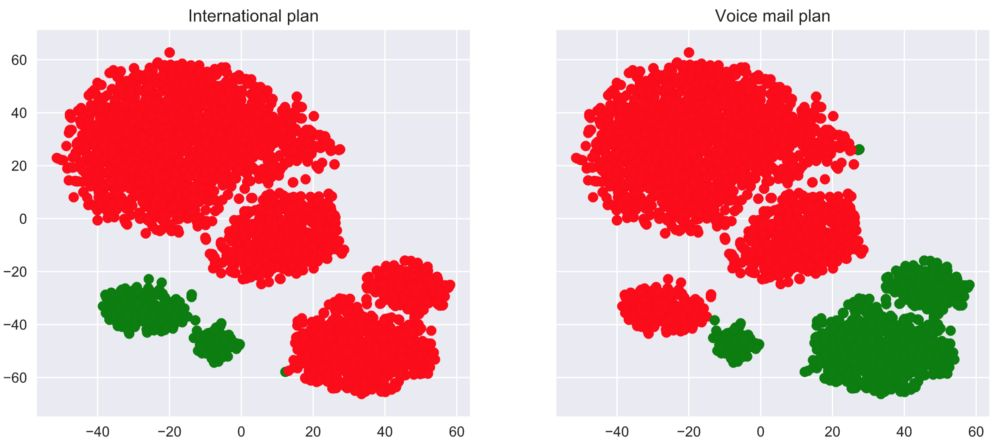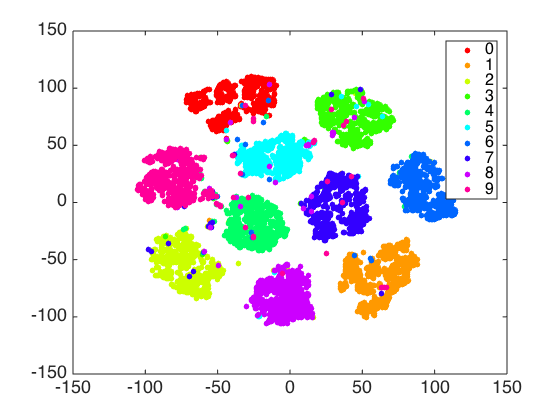• 机器人
+关注

关注

201

文章

23962

浏览量

197357
+关注

关注

5

文章

1643

浏览量

56471
• 数据集
+关注

关注

4

文章

890

浏览量

23969
• 开发编程
+关注

关注

0

文章

4

浏览量

5703

相关推荐

## 深圳泰科智能机械臂RJS14机器人关节模组

机器人具备哪些优势？ 一、编程简单可以让无编程经验的操作人员能快速设置和操作我们的直观、3D 可视化协作式机器人。只需将机器人手臂移动至需要的位置，或触摸方便易用的触屏
发表于 04-19 15:13

## 自己开发的机器人图形化编程软件

开发机器人图形编程软件从2005年起就开始搞机器人教学了，至今每年夏天都举行机器人夏令营。10年来一直尝试做一款简单稳定好用的机器人编程软件，上次做的类似于app inventor 的积木
发表于 07-02 20:15

## 可视化机器人

机器人配有两个直流电机作为动力来源，采用履带式行进，机器人上面有四个摆臂，可以自由灵活摆动。我们给该机器人开发有相应的上位机，和控制手柄。该机器人有三中控制模式：通过手柄控制的手动控制模式，通过
发表于 07-31 13:14

## 采用MATLAB设计下的可视化与控制

发表于 05-21 09:38

## 数据可视化之Python-matplotlib概述

数据可视化（二）：Python-matplotlib
发表于 07-22 14:58

## python数据可视化的方法和代码

Python数据可视化汇总
发表于 10-14 14:59

## Python数据可视化专家的七个秘密

Python数据可视化专家的七个秘密
发表于 05-15 06:43

## python数据可视化之画折线图

python数据可视化之画折线图，散点图
发表于 05-27 08:09

## Python数据可视化

Python数据可视化：网易云音乐歌单
发表于 07-19 08:30

## 可视化编程LCD模组技术特性是什么？

可视化编程LCD模组技术特性是什么？在编写单片机AT89C2051的控制软件时需注意有哪些事项？
发表于 06-08 06:13

## 编程0基础他们，是这样掌握数据可视化的

编程基础，能不能掌握数据可视化？”事实上，用户没有编程基础也没什么影响，照样能把数据可视化做得又快又直观。废话少说，看看他们是怎么从编程0基础开始掌握数据可视化的。编程0
发表于 07-08 11:36

## 机器人路线规划

Python编程画个图，想输出这个图形每一段路线的向量，然后可以设置运动时间来控制机器人运动的距离就是我怎么用labview去调用这个Python
发表于 11-18 16:21

## 如何设计液压式双足机器人运动数据可视化系统

机器人由于具有高负载、快速动态响应等优点，逐渐地应用于军事、救灾等复杂危险的任务中。设计一个稳定、高效的运动数据感知获取系统并实现数据可视化显示，对双足机器人的步态规划、运动控制以及运动状态
发表于 03-19 16:03 6次下载## Python数据可视化编程实战

Python数据可视化编程实战资料免费下载。
发表于 06-01 14:37 28次下载

## Python拉勾网数据采集与可视化

数据，采集的是Python岗位的数据，然后用Python进行可视化。主要涉及的是爬虫&数据可视化的知识。发表于 03-13 14:18 2722次阅读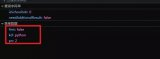### 下载硬声App